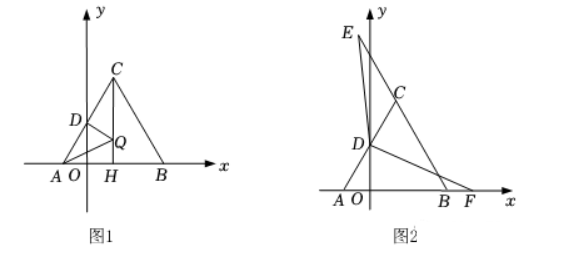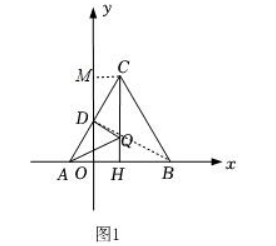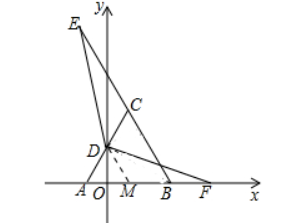(1) 求 $m, n$ 的值;
(2) 如图1, 过点 $\mathrm{C}$ 作 $\mathrm{CH} \perp \mathrm{AB}$, 垂足为点 $\mathrm{H}$, 且 $\mathrm{CH}=2$, 当动点 $\mathrm{Q}$ 在线段 $\mathrm{CH}$ 上运动时, 请你求 出 $\mathrm{AQ}+\mathrm{DQ}$ 的最小值;
(3) 如图2, 点 $E$ 在线段 $B C$ 的延长线上, 点 $F$ 在线段 $A B$ 的延长线上, 当 $\angle E D F=120^{\circ}$ 时, 请问 $\mathrm{CE}-\mathrm{BF}$ 的值是否发生变化? 若改变, 请说明理由; 若不变, 请求出CE-BF的值.【答案】 解 :
(1 ) $\because m^2+n^2+2 m-6 n+10=0$,
\begin{aligned} & \therefore m^2+2 m+1+n^2-6 n+9=0 \\ & \therefore(m+1)^2+(n-3)^2=0, \\ & \therefore m+1=0, n-3=0, \\ & \therefore m=-1, n=3 ; \end{aligned}

（2）
\begin{aligned} \because m=-1, n=3, \\ & \therefore A(-1,0), B(3,0), \\ & \therefore A B=4, \\ & \therefore A H=2, \\ & \therefore O H=1, \\ & \therefore C(1,2 \sqrt{3}), \end{aligned}\begin{aligned} & \because C M=O A=1, \angle C M D=\angle A O D=90^{\circ}, \angle C D M=\angle A D O, \\ & \therefore \triangle C D M \cong \triangle A D O(A A S), \\ & \therefore C D=A D, \end{aligned}

\begin{aligned} & \because C H=2 \sqrt{3}, \\ & \therefore B D=2 \sqrt{3}, \\ & \therefore A Q+D Q \text { 的最小值为 } 2 \sqrt{3} \text {; } \end{aligned}

(3) $C E-B F$ 的值不会发生变化.

$$\therefore A D=C D=A M=2 \text {, }$$\begin{aligned} & \because \angle E D F=\angle C D M=120^{\circ}, \\ & \therefore \angle E D C=\angle M D F, \\ & \because C D=A D=D M, \angle D C E=\angle D M F=120^{\circ}, \\ & \therefore \triangle D C E \cong \triangle D M F(A S A), \\ & \therefore E C=M F, \\ & \therefore E C-B F=M F-B F=M B=A M=2 . \end{aligned}